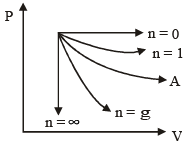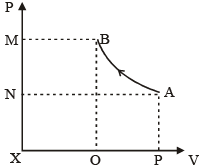Test: Thermodynamics- 1

# Test: Thermodynamics- 1 - Mechanical Engineering

Test Description

## 25 Questions MCQ Test Mechanical Engineering SSC JE (Technical) - Test: Thermodynamics- 1

Test: Thermodynamics- 1 for Mechanical Engineering 2023 is part of Mechanical Engineering SSC JE (Technical) preparation. The Test: Thermodynamics- 1 questions and answers have been prepared according to the Mechanical Engineering exam syllabus.The Test: Thermodynamics- 1 MCQs are made for Mechanical Engineering 2023 Exam. Find important definitions, questions, notes, meanings, examples, exercises, MCQs and online tests for Test: Thermodynamics- 1 below.
Solutions of Test: Thermodynamics- 1 questions in English are available as part of our Mechanical Engineering SSC JE (Technical) for Mechanical Engineering & Test: Thermodynamics- 1 solutions in Hindi for Mechanical Engineering SSC JE (Technical) course. Download more important topics, notes, lectures and mock test series for Mechanical Engineering Exam by signing up for free. Attempt Test: Thermodynamics- 1 | 25 questions in 50 minutes | Mock test for Mechanical Engineering preparation | Free important questions MCQ to study Mechanical Engineering SSC JE (Technical) for Mechanical Engineering Exam | Download free PDF with solutions
 1 Crore+ students have signed up on EduRev. Have you?
Test: Thermodynamics- 1 - Question 1

### Which of the following is an intensive property ?

Test: Thermodynamics- 1 - Question 2

### Work and heat are 1. Point functions 2. Path functions 3. Exact Differential 4. Inexact Differential

Test: Thermodynamics- 1 - Question 3

### What is mean free path ?

Test: Thermodynamics- 1 - Question 4

Which of the following are pure substance.
i.O2 & N2 mixture
ii. Moist Air
iii. Dry Air.

Test: Thermodynamics- 1 - Question 5

When water is at saturated condition (i.e.Saturated liquid or saturated vapour) no. of independent intensive properties required to determine the state is

Test: Thermodynamics- 1 - Question 6

Degree of freedom at tripple point of water is

Test: Thermodynamics- 1 - Question 7

The thermometric property in a thermometer is

Test: Thermodynamics- 1 - Question 8

The steam point is 100º C or 212F. The Ice point is 0ºC or 32ºF.The relationship b/w  ºC and ºF is

Test: Thermodynamics- 1 - Question 9

It E = Energy Transfer M = Mass transfer In a isolated system which of the following is true,

Test: Thermodynamics- 1 - Question 10

An ideal gas at 127ºC is heated till its volume becomes two times at constant pressure. The new temperature is

Test: Thermodynamics- 1 - Question 11

Pressure of a system is said to be zero when

Test: Thermodynamics- 1 - Question 12

In a new temperature scale say of the boiling and freezing point of water at one atmosphere are  100º f and 300º f respectively, corelate this scale with centrigate scale. The reading of 0º f on the centrigate scale is

Test: Thermodynamics- 1 - Question 13

The pressure and temperature of tripple point of water is :

Test: Thermodynamics- 1 - Question 14The process marked A is :

Test: Thermodynamics- 1 - Question 15

The diagram below shows a compression process in P-V diagram. Work required by a centrifugal compressor is equal to the areaTest: Thermodynamics- 1 - Question 16

A container contains mixure of O2 gas and N2 gas. The number of independent intrinsic variables required to completely define The state of the system is

Test: Thermodynamics- 1 - Question 17

Change in internal energy in a reversible process occuring in a closed system is equal to the heat transfered in the process occurs at constant

Test: Thermodynamics- 1 - Question 18

A water heater of power 2kw is switched on for 10 minutes. The capacity of heater is 10 liter of water . If heat capacity of water is 4 KJ/KgK.The rise in temperature is :

Test: Thermodynamics- 1 - Question 19

In a isothermal process the fluid expands from 5 bar and 2m3 to 2.5 bar and 4m3. The heat added during the process is 50 kJ. The net work done during the process is.

Test: Thermodynamics- 1 - Question 20

In a thermal process, the pressure of a fixed mass of a gas is changed in such a manner that the gas molecules give out the heat of 30 J and work of 10 J is done on the gas. If the initial internal energy of the gas was 40 J, then the final internal energy will be?

Detailed Solution for Test: Thermodynamics- 1 - Question 20

∆Q = (Uf - Ui) + ∆W
30 = Uf - 40 + 10
Uf = 60J.

Test: Thermodynamics- 1 - Question 21

A real gas obeys perfect gas laws at very.
1. Low temperatures
2. high temperatures
3. Low pressures
4. High pressures

Test: Thermodynamics- 1 - Question 22

A heat engine works on carnot cycle work output is half of heat rejected to sink. The efficiency of the engine is

Test: Thermodynamics- 1 - Question 23

A Carnot cycle works between 27 ºC and 327 ºC. If engine produces 100 kJ of work  the entropy change during heat rejection is .

Test: Thermodynamics- 1 - Question 24

1. An adiabatic process is always a constant entropy process
2. In an adiabatic process heat transfer is equal to zero.

Test: Thermodynamics- 1 - Question 25

the dimentions of entropy is (q - Temperature)

## Mechanical Engineering SSC JE (Technical)

6 videos|97 docs|57 tests
Information about Test: Thermodynamics- 1 Page
In this test you can find the Exam questions for Test: Thermodynamics- 1 solved & explained in the simplest way possible. Besides giving Questions and answers for Test: Thermodynamics- 1, EduRev gives you an ample number of Online tests for practice

## Mechanical Engineering SSC JE (Technical)

6 videos|97 docs|57 tests